Test: Probability & Statistics- 1

# Test: Probability & Statistics- 1

Test Description

## 20 Questions MCQ Test GATE Computer Science Engineering(CSE) 2023 Mock Test Series | Test: Probability & Statistics- 1

Test: Probability & Statistics- 1 for Civil Engineering (CE) 2023 is part of GATE Computer Science Engineering(CSE) 2023 Mock Test Series preparation. The Test: Probability & Statistics- 1 questions and answers have been prepared according to the Civil Engineering (CE) exam syllabus.The Test: Probability & Statistics- 1 MCQs are made for Civil Engineering (CE) 2023 Exam. Find important definitions, questions, notes, meanings, examples, exercises, MCQs and online tests for Test: Probability & Statistics- 1 below.
Solutions of Test: Probability & Statistics- 1 questions in English are available as part of our GATE Computer Science Engineering(CSE) 2023 Mock Test Series for Civil Engineering (CE) & Test: Probability & Statistics- 1 solutions in Hindi for GATE Computer Science Engineering(CSE) 2023 Mock Test Series course. Download more important topics, notes, lectures and mock test series for Civil Engineering (CE) Exam by signing up for free. Attempt Test: Probability & Statistics- 1 | 20 questions in 60 minutes | Mock test for Civil Engineering (CE) preparation | Free important questions MCQ to study GATE Computer Science Engineering(CSE) 2023 Mock Test Series for Civil Engineering (CE) Exam | Download free PDF with solutions
 1 Crore+ students have signed up on EduRev. Have you?
Test: Probability & Statistics- 1 - Question 1

### A fair dice is rolled twice. The probability that an odd number will follow an even number is

Detailed Solution for Test: Probability & Statistics- 1 - Question 1

Here the sample space S = 6

Therefore  P(odd number ) = 3/6 = 1/2

and  P (even number )  = 3/6 = 1/2

since events are independent,

therefore, P (odd / even )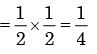Test: Probability & Statistics- 1 - Question 2

### Three companies X, Y and Z supply computers to a university. The percentage of computers supplied by them and the probability of those being defective are tabulated below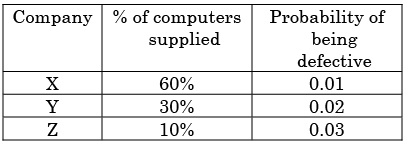Given that a computer is defective, the probability that it was supplied by Y is

Detailed Solution for Test: Probability & Statistics- 1 - Question 2

Probability of defective computer supplied by Y =
(Case when Y produces defective)/(All cases of producing defective product)
Case when Y produces defective = (0.3)(0.02) = 0.006
All cases of producing defective product= (0.6x0.01)+(0.3x0.02)
(0.1x0.03)= 0.006+0.006+0.003=0.015

Probability = 0.006/0.015=0.4

Test: Probability & Statistics- 1 - Question 3

### An examination consists of two papers, Paper 1 and Paper 2. The probability of failing in Paper 1 is 0.3 and that in Paper 2 is 0.2. Given that a student has failed in Paper 2, the probability of failing in Paper 1 is 0.6. The probability of a student failing in both the papers is

Detailed Solution for Test: Probability & Statistics- 1 - Question 3

Let A be  the event that ‘failed in paper 1’.

B be the event that ‘failed  in paper 2’.

Given P(A) = 0.3, P(B) = 0.2.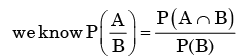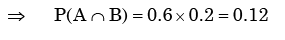Test: Probability & Statistics- 1 - Question 4

A fair coin is tossed 10 times. What is the probability that ONLY the first two tosses will yield heads?

Detailed Solution for Test: Probability & Statistics- 1 - Question 4

Let A be the event that first toss is head

And B be the event that second toss is head.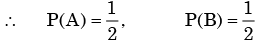By the given condition rest all 8 tosses should be tail

∴ The probability of getting head in first two cases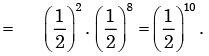Test: Probability & Statistics- 1 - Question 5

Consider the continuous random variable with probability density function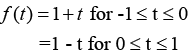The standard deviation of the random variables is

Detailed Solution for Test: Probability & Statistics- 1 - Question 5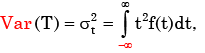T being the random variable of f(t).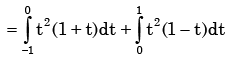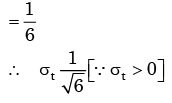Test: Probability & Statistics- 1 - Question 6

The probability that two friends share the same birth-month is

Detailed Solution for Test: Probability & Statistics- 1 - Question 6

Let A = the event that the birth month of first friend

And B= that of second friend.

∴ P( A )= 1, as 1st friend can born in any month

and P(B) = 1/12, by the condition.

∴ Probability of two friends share same birth-month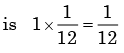Test: Probability & Statistics- 1 - Question 7

A box contains 5 block balls and 3 red balls. A total of three balls are picked from the box one after another, without replacing them back. The probability of getting two black balls and one red ball is

Detailed Solution for Test: Probability & Statistics- 1 - Question 7

Here the possible combination of picking up three balls without replacement is  BBR,  BRB,  RBB.

(B = Black ball,  R = Red balls)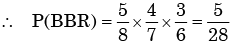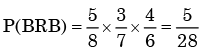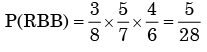∴ Probability of getting two black balls and one red ball is 5/28.

Test: Probability & Statistics- 1 - Question 8

Two dice are thrown. What is the probability that is the sum of the numbers on the two dice is eight?

Detailed Solution for Test: Probability & Statistics- 1 - Question 8

Here sample space = 6 × 6 = 36

Here, there are five such points whose sum is 8. They are (2,6), (3,5), (4,4), (5,3), (6,2).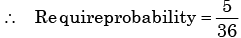Test: Probability & Statistics- 1 - Question 9

Arrivals at a telephone booth are considered to be poison, with an average time of 10 minutes between successive arrivals. The length of a phone call is distributes exponentially with mean 3 minutes. The probability that an arrival does not have to wait before service is

Detailed Solution for Test: Probability & Statistics- 1 - Question 9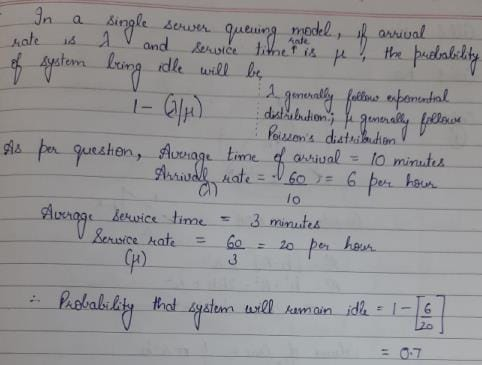Test: Probability & Statistics- 1 - Question 10

A box contains 5 black and 5 red balls. Two balls are randomly picked one after another from the box, without replacement. The probability for both balls being red is

Detailed Solution for Test: Probability & Statistics- 1 - Question 10

The probability of drawing two red balls without replacement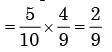Test: Probability & Statistics- 1 - Question 11

A lot has 10% defective items. Ten items are chosen randomly from this lot. The probability that exactly 2 of the chosen items are defective is

Detailed Solution for Test: Probability & Statistics- 1 - Question 11

Let A be the event that items are defective and B be the event that items are non- defective

∴ P( A )= 0.1 and P(B) = 0.9

∴ Probability that exactly two of those items are defective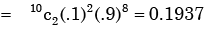Test: Probability & Statistics- 1 - Question 12

A box contains 20 defective items and 80 non-defective items. If two items are selected at random without replacement, what will be the probability that both items are defective?

Detailed Solution for Test: Probability & Statistics- 1 - Question 12

The probability of defective  items = 20/100

Therefore the probability of first two defective items without replacement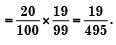Test: Probability & Statistics- 1 - Question 13

If three coins are tossed simultaneously, the probability of getting at least one head is

Detailed Solution for Test: Probability & Statistics- 1 - Question 13

Here the sample space S =23= 8.

No. of ways to get all tails =1.

∴ probability to get all tails = 1/8

∴ Probability to get at least one head is = 1-1/8= 7/8

Test: Probability & Statistics- 1 - Question 14

If 20 per cent managers are technocrats, the probability that a random committee of 5 managers consists of exactly 2 technocrats is

Detailed Solution for Test: Probability & Statistics- 1 - Question 14

The probability of technocrats manager = 20/100 = 1/5

∴ Probability of non technocrats manager = 4/5

Now the require probability  ​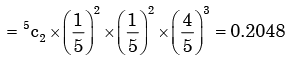Test: Probability & Statistics- 1 - Question 15

Four arbitrary point (x1,y1), (x2,y2), (x3,y3), (x4,y4), are given in the x, y – plane Using the method of least squares, if, regressing y upon x gives the fitted line y = ax + b; and regressing y upon x given the fitted line y = ax + b; and regressing x upon y gives the fitted line x = cy + d then

Detailed Solution for Test: Probability & Statistics- 1 - Question 15

y =ax+b − (i) and x = cy + d − (ii)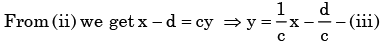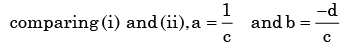Test: Probability & Statistics- 1 - Question 16

Chebyshev’s inequality states that the probability of a “Six Sigma” event is less than :

Detailed Solution for Test: Probability & Statistics- 1 - Question 16

If a bell curve is assumed, the probability of a “six sigma” event is on the order of one ten millionth of a percent.

Test: Probability & Statistics- 1 - Question 17

Three values of x and y are to be fitted in a straight line in the form y = a + bx by the method of least squares. GivenΣx = 6, Σy = 21, Σx2 = 14 and Σxy = 46, the values of a and b are respectively.

Detailed Solution for Test: Probability & Statistics- 1 - Question 17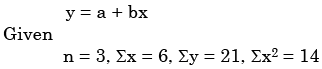​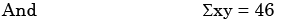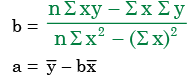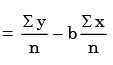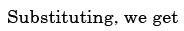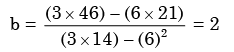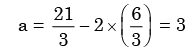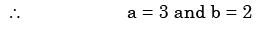Test: Probability & Statistics- 1 - Question 18

A box contains 10 screws, 3 of which are defective. Two screws are drawn at random with replacement. The probability that none of the two screws is defective will be

Detailed Solution for Test: Probability & Statistics- 1 - Question 18

A = First drawn screw non-defective.

B = Second drawn screw non-defective.

P(A) = 7/10

Because 7 out of 10 screws are non-defective and we sample at random,

P(B) = 7/10

The second drawing is the same as the beginning.

P(A Intersection B) = P(A) * P(B) = 0.7 * 0.7 = 0.49

It is Converted to percentage = 0.49 * 100 = 49%

The probability of two screws are defective is 49%.

Test: Probability & Statistics- 1 - Question 19

Which one of the following statements is NOT true?

Test: Probability & Statistics- 1 - Question 20

If the standard deviation of the spot speed of vehicles in a highway is 8.8 kmph and the mean speed of the vehicles is 33 kmph, the coefficient of variation in speed is

Detailed Solution for Test: Probability & Statistics- 1 - Question 20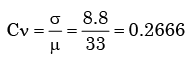## GATE Computer Science Engineering(CSE) 2023 Mock Test Series

150 docs|215 tests
 Use Code STAYHOME200 and get INR 200 additional OFF Use Coupon Code
Information about Test: Probability & Statistics- 1 Page
In this test you can find the Exam questions for Test: Probability & Statistics- 1 solved & explained in the simplest way possible. Besides giving Questions and answers for Test: Probability & Statistics- 1, EduRev gives you an ample number of Online tests for practice

## GATE Computer Science Engineering(CSE) 2023 Mock Test Series

150 docs|215 tests